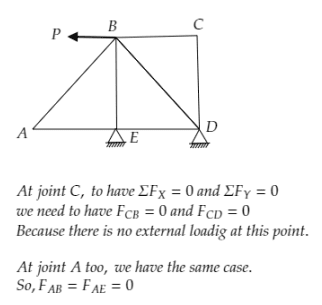Search
• APSEd

# How to find zero force members with zero effort in a truss?

Updated: Dec 1, 2020

Zero force members reduce our unknown force calculation in a truss. There are two reasons for putting a zero force member in the truss. Either to increase the stability or there may be a change in loading with due course of time.

Zero-force members is a commonly asked question in exams and a MUST know in your exam preparation.## What is a zero-force member?

To find out these zero force members, we have some rules. By simple inspection, we can find these out. In a truss, all members carry only axial force. If in a member, the axial force is zero - it is known as zero force member.

For truss analysis, we use the following two methods - Method of Joints and Method of Section

### Method of joints

For any truss joint, the summation of horizontal and vertical forces are zero.

But this method is of no use when the structure is of big size and we need member force at any of the sections.

### Method of sections

It uses all three static equilibrium equations. But we should cut the section in such a way that, there are at most three unknown forces/members are there

Now, let us see how we can identify them## Rules to find zero force member in a truss

There are two basic rules which you can apply to find the zero-force members.

### Rule 1

If two non-collinear members meet at a joint and there is no external loading then from the joint equilibrium there should be no force in both the members.

### Rule 2

If three members form a joint and two of them are collinear then the third member will be a zero force member and the rest two members carry equal and opposite force, provided there is no external load acting at the joint.

Let us get in-depth and clear it. Watch the video and you will never have to worry again.

Were you able to understand?

### Try this!

Any questions put it down in the comments section.